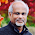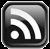# LEARN MS-ACCESS TIPS AND TRICKS

Learn Microsoft Access Advanced Programming Techniques, Tips and Tricks.

### Introduction

MS-Access has Date/Time Data Field Type to store Date or Time or both Date and Time together.  When you enter a Date Value into the Field (such as 14/07/2010) the actual value stored in memory is a whole number 40373, the number of days from 30-12-1899.  Day 1 is 31-12-1899.

You can easily find this if you type? format(1, "dd/mm/yyyy") in the Debug Window (Alt+F11 to display VBA Editing Window and Ctrl+G to show Debug Window) and press Enter Key to show the result value 31-12-1899.

Like Date; Time is internally stored as a Decimal Number. Time at midnight is 0.0 and 0.5 at 12 Noon. So the Date and Time Value combined on 14th July 2010 at 12 Noon is 40373.5.

To find the difference in time between Midnight and a time value before that, the midnight value will be taken as 24.00 for calculation purposes instead of 0.00.

Type ? format(40373.5,"dd/mm/yyyy hh:nn:ss") and press Enter Key.

Result:  14/07/2010 12:00:00

It is interesting to explore how 0.5 becomes 12:00:00 noon or how the System maintains Date and Time internally?

We know we have 24 Hours in a Day or 24 x 60 = 1440 minutes in a Day or 24 x 60 x 60 = 86400 Seconds in a Day.

### Time Calculations

That is 1 Second = 1 Day/86400 Seconds = 0.000011574074074074 Day (we can take it rounded as 0.0000115741).  The end value of 074 is infinite.

From Midnight the Value 0.0000115741 (equal to one Second) is added to the Time of the Day Value at every one-second interval. One Second before midnight (23:59:59 Hrs.) the value is 0.9999906659 (86399 Seconds) and after one second; a Day is added to the Date Value.  So at Midnight of 14/07/2010, the Number of Days becomes 40374.

But, each Second is further divided into Milliseconds and this can be read with the use of the Timer Built-in Function.

For example, type the following direct command in the Debug Window:

? Timer

You will get the output something like the example given below depending on the time you try out this.

Result in Seconds: 68473.81

The Value .81 part is the time in milliseconds and 68473 the number of seconds of the current time of the day.

If you want to see this value in Current Time of the Day format type the following expression in Debug Window and press Enter Key:

? format(68473.81/86400,"hh:nn:ss")

OR

? format(68473.81*0.0000115741,"hh:nn:ss")

The Value of 68473.81 Seconds is converted into its equal value in Days by multiplying it with 0.0000115741.

Result: 19:01:14

### Using the Timer Function.

You can use the Timer() Function to build a delay loop in Program, like the example given below, to slow down some action in Programs.

```Public Function myFunction()
.
.
.
t = Timer
Do While Timer < t + 5

DoEvents

Loop
.
.
.
End Function
```

The sample program segment above slows down the action by 5 Seconds before executing the next statement after the Loop statement.

We can get the current Date and Time Value from the System with the use of the Now() Built-in Function.  The Date() Function returns the Current System Date.

While designing a Table you can set the Default Value Property of the Date/Time Field to Date() Function or Now() Function to insert Current System Date or Current Date and Time Stamp respectively, when a new record is added to the Table.

Since we have learned the basics of the internal representation of Time Values, let us look into few examples of Normal Time Value conversions of Hours, Minutes, and Seconds.

Always use the Date and Time Values together to calculate and find time differences properly. While designing Tables, if the time involves in any calculations then store Date and Time values together in the Date/Time Field. Not to save Date and Time in separate Fields.  This is very important if the time period is extended to more than one day like work starts at 20:00 hrs and ends at 04:00 hrs the next day.

Assume that we have Date and Time values separately, like 25/10/2020, and time value 5 Hours 7 Minutes, and 15 Seconds.  How do we convert these values to the internal storage format along with the date?

Date and Time Converting to store in Date/Time Field.

The Date Number of 25/10/2020 is 44129 in the Integer form.

To cross-check whether the number is correct or not, type the following expression in the VBA Debug window and print the result:

```? format(#25/10/2020#,"0")

Result: 44129
```

Now, all the time values (5 Hours, 7 Minutes, and 15 Seconds) we need to convert into seconds first then add them all together and divide the result by 86400 or (24*60*60) to get the internal time format suitable to add to the date number so that date and time value stays together in the Date/Time Field.

Now let us do that as follows:

```d_date = #25/10/2020#

hrs = 5
min = 7
sec = 15

h_seconds = hrs * 60 * 60
m_seconds = min * 60
Total = h_seconds + m_seconds + sec
? Total
Result: 18435 'seconds

'Convert to Time Value
timVal = Total/86400
OR
timval = Total/(24*60*60)

? timval
Result: 0.213368055555556

d_date = d_date + timval
'Print the value of d_date in Date/time format
? format(d_date,"dd/mm/yyyy hh:nn:ss")

Result: 25/10/2020 05:07:15

```

You may convert the Hours, Minutes, and Seconds into Time Value format in a single expression:

```timval = (((hrs*3600)+(min*60)+sec)/86400)d_date = d_date + timval
OR
d_date = d_date + (((hrs*3600)+(min*60)+sec)/86400)
```

Date/Time Values change to Date, Hours, Minutes, and Seconds.

How do we separate again into Date, Hours, Minutes, and Seconds, if we want them in that way again from the Date/Time Values?

```'The Date/Time Value
'we have the date+time in:
d_date = d_date + timval

'get date value separate
dt = int(d_date)

timval = d_date - dt

'get hours
hrs = int(timval*24)

'subtract hrs value from time value
timval = timval - ((hrs*3600)/86400)

'get Minutes
min = int(timval * (24*60))

'subtract Minutes from time value
timval = timval-(min*60/86400)

'get seconds
s = int(timval*86400+0.1)
```

The +0.1 added for correction of rounding Error of actual value of

The Simple Recommended Method.

If you want to do it differently here it is:

```d = 1/86400 :'1 second value = in day value internaly
H = 5
M = 7
S = 15

t = ((H*3600)+(M*60)+S)/86400? t0.213368055555556
TotalSeconds = t/d

? TotalSeconds
18435

hr = int(TotalSeconds/3600)
? hr
5
bal = TotalSeconds-(hr*3600)
? bal
435
mi = int(bal/60)
? mi
7
se = bal-(mi*60)
? s
15
?
? format(t,"hh:nn:ss")
05:07:15
```

You can follow any method you feel comfortable working with and I recommend the last one.

Share:

1.[...] Quarter:GetQrtr(Month([Visit Date])) Find out how date and time values are stored internally from here. __________________ http://www.msaccesstips.com (Learn MS-Access Tips and Tricks) Learn [...]

2.Converting Date information currently in a text file in YYYY-MM-DD format into an MS-Access compatible date format MM/DD/YYYY.

Does anyone have a suggestion on how to do this?

3.Example:
Dt = "2020-10-31"
? DateValue(Dt)
Result: 31-10-2020
OR
x = dateserial(left(dt,4),mid(dt,6,2),right(dt,2))
? x
Result: 31-10-2020

Change the Regional Settings of Windows Date/Time Format to change the result in American Date Format: 10-31-2020

Comments subject to moderation before publishing.

## FeedSubscribe in a readerYour email address:

Delivered by FeedBurner